## Friday, January 8, 2016

### Alternative density tree plotting method in phytools (that also allows stochastic map trees)

A few of days ago, an R-sig-phylo subscriber asked if there was a way to represent the distribution of trees in a single plot in a manner “graphical style similar to the phytools::fancyTree(type = "phenogram95”)“.

In fact, such a function (`densitTree`) exists in phangorn; however since the subscriber (Giulio Dalla Riva at the University of Canterbury) added the challenge "bonus point if anybody proposes a solution that works with plotSimmap for painted trees”, I thought it might be worth demonstrating how this can be adapted to work with phytools plotting functions such as `plotTree` or `plotSimmap`.

I sent some code to the list, but it is fairly straightforward to write a custom function that does this visualization:

``````make.transparent<-function(color,alpha){
RGB<-col2rgb(color)[,1]/255
rgb(RGB,RGB,RGB,alpha)
}

density.tree<-function(trees,colors="blue",alpha=NULL,method="plotTree",fix.depth=FALSE,...){
N<-length(trees)
if(hasArg(use.edge.length)) use.edge.length<-list(...)\$use.edge.length
else use.edge.length<-TRUE
if(!use.edge.length) trees<-lapply(trees,compute.brlen)
if(!fix.depth){
h<-sapply(trees,function(x) max(nodeHeights(x)))
ii<-order(h,decreasing=TRUE)
trees<-trees[ii]
h<-h[ii]
}
if(is.null(alpha)) alpha<-1/N
colors<-setNames(sapply(colors,make.transparent,alpha),names(colors))
if(method=="plotTree") foo<-plotTree else foo<-plot
foo(trees[],color=colors,...)
xlim<-get("last_plot.phylo",envir=.PlotPhyloEnv)\$x.lim
xlim<-xlim+0.03703704*diff(xlim)
xlim<-xlim-0.03703704*diff(xlim)
par(fg="transparent")
for(i in 2:length(trees))
foo(trees[[i]],
tips=setNames(1:Ntip(trees[]),trees[]\$tip.label),
xlim=if(fix.depth) NULL else xlim-(h-h[i]),...)
par(fg="black")
}
``````

First, let's use the function to do a basic visualization of topological and branch length uncertainty:

``````library(phytools)
density.tree(trees,lwd=3,ftype="i",fsize=0.9)
``````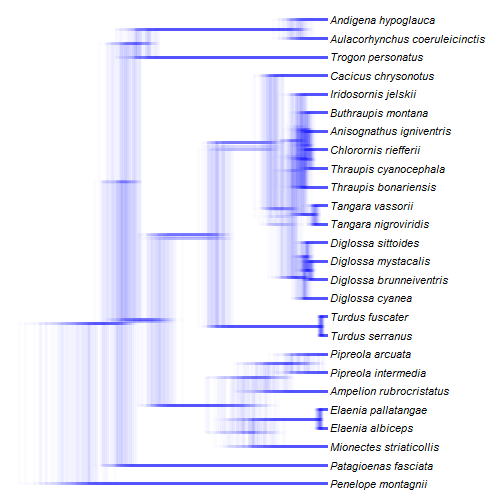We have all the options of `plotTree` & `plotSimmap` at our disposal here, and I find this type of visualization more readable with a non-standard node position which in phytools is called `nodes="inner"`:

``````density.tree(trees,lwd=3,ftype="i",fsize=0.9,nodes="inner")
``````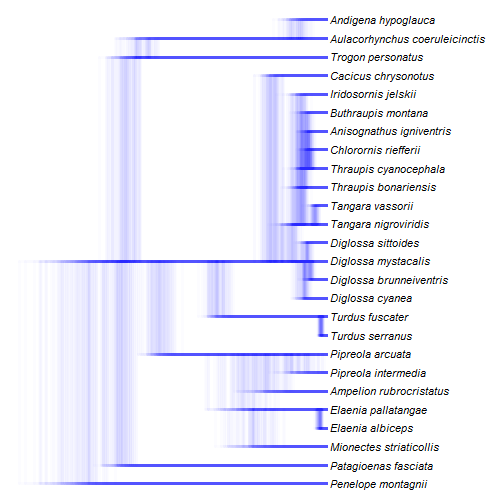In this visualization, trees have variable depth (because the input trees were variable in total depth, but we can also turn this off and plot the trees with a standardized depth:

``````density.tree(trees,lwd=3,colors="black",ftype="i",fsize=0.9,nodes="inner",fix.depth=TRUE)
``````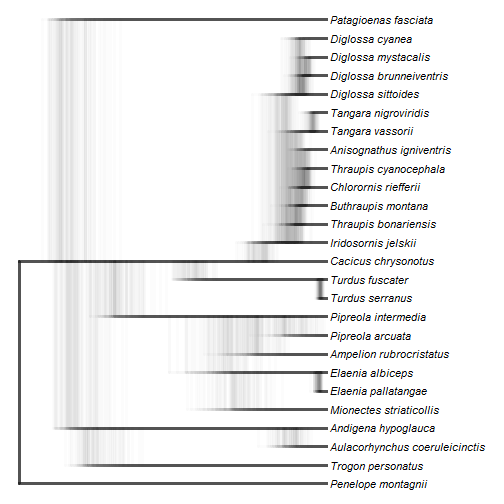Finally, we can also turn off edge lengths entirely for this basic visualization by setting `use.edge.length=FALSE`:

``````density.tree(trees,lwd=3,ftype="i",fsize=0.9,nodes="inner",use.edge.length=FALSE)
``````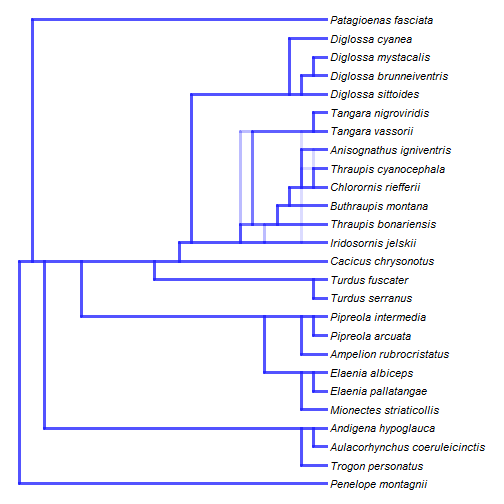As noted above, it is fairly straightforward to switch the plotting method used internally by this function to `plotSimmap` and thus plot stochastic map-style painted trees. For this example I will just use some simulated data:

``````Q<-matrix(c(-0.02,0.02,0.02,-0.02),2,2)
rownames(Q)<-colnames(Q)<-letters[1:2]
x<-sim.history(trees[],Q)\$states
``````
``````## Done simulation(s).
``````
``````mtrees<-make.simmap(trees,x,message=FALSE)
mtrees
``````
``````## 100 phylogenetic trees with mapped discrete characters
``````
``````density.tree(mtrees,colors=setNames(c("red","blue"),sort(unique(x))),
nodes="inner",ftype="i",lwd=5,method="plotSimmap")
prompt=FALSE,x=par()\$usr+8,y=par()\$usr-1)
``````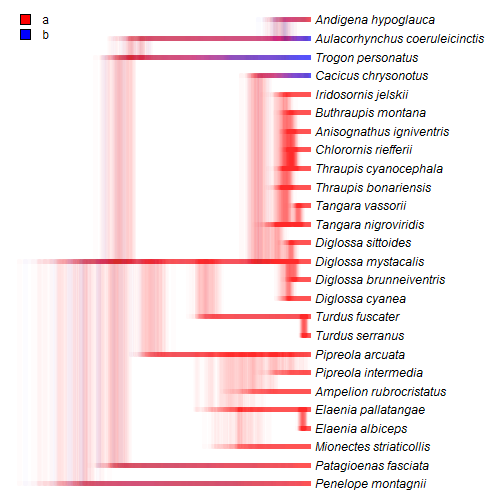``````## or
density.tree(mtrees,colors=setNames(c("red","blue"),sort(unique(x))),
nodes="inner",ftype="i",lwd=5,method="plotSimmap",fix.depth=TRUE)
prompt=FALSE,x=par()\$usr+1,y=par()\$usr-1)
``````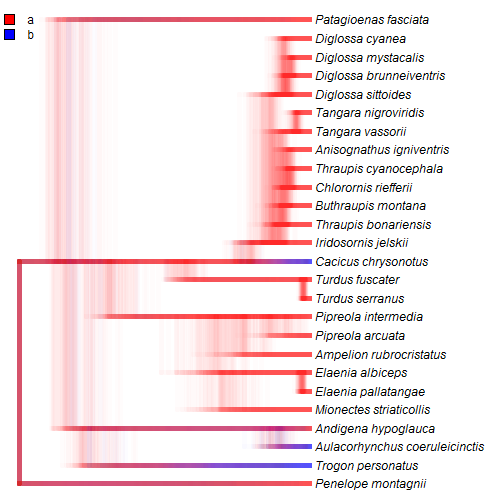That's it really.

One feature that would be good to include is a preliminary calculation of the tip order in a consensus tree. Presently, the tip order is the order of the deepest tree - which is fine when the trees vary little in topology, as in this case, but will work poorly if that tree happens to be quite topologically different from the rest of the trees in the sample.